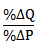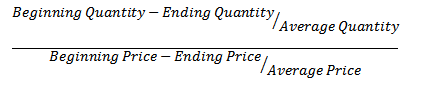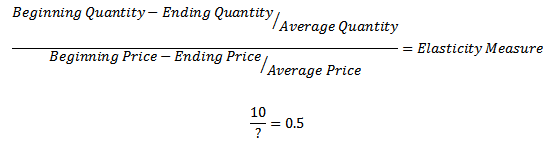How to solve elasticities problems in economics - FreeEconHelp.com, Learning Economics... Solved!

## 5/29/11

Calculating elasticities is actually very easy.  You just need to know several of the values, then plug them into their proper place.The first equation is for the price elasticity of demand.  In order to calculate this, we need the beginning and ending price, and the beginning and ending quantity.  By beginning and ending, I mean before and after the price/quantity change.Here just plug in the values given to their appropriate spot.  Some students get confused about which price/quantity goes first, but actually it does not matter as long as you are consistent.  Try it with some example values and you will see that subtracting ending from beginning or vice verse has no impact on the final answer.

If you get a question that gives you the elasticity measurement and wants you to solve for one of the price or quantity levels, this is also easy to solve for given some algebraic manipulation.Divide both sides by 0.5 and multiply both sides by ? to end up with 20=?, so we know that the percent change in price had to be 20, in order to get the elasticity measure of 0.5.

Another example:
Suppose the price elasticity of demand coefficient for the product of a firm is 2,0 (ignore negative sign) the firm decides to increase the price of its product from 600 to 612. if the firm is currently selling 1500 units per month, how many will be sold after the price increase?

To get this number we have to figure out what the % change in price is first: 612-600/600 =  .02% change.  Now we know that the elasticity of demand is 2.0, so we can multiply our elasticity measure by the % change in price to get our % change in quantity, remember:

% change in quantity / % change in price = elasticity

we can rearrange this to get:

elasticity * % change in price = % change in quantity

or for our problem:

2 * 0.02 = 0.04

We now have to change the quantity by -4 percent, or mutiply 1500 by 96% (100-4) to get:
1500*.96=1440

So the quantity sold after the price increase goes down by 60.  Also note that total revenue has declined.  Click here for more info on the relationship between revenue and elasticities.